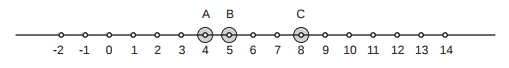### I - Ingenious Metro

##### Languages: C, C++, Java, JavaScript, ... (details)

The King of Logonia will inaugurate soon a new and revolutionary metro, based on an invention of the Royal Engineers, which allows teletransportation.

The new metro consists of a very long tunnel with a station at each kilometer. There are also $T$ teletransporters, which are located at some of the stations. In each station there is a keyboard with $T$ keys, where each key corresponds to one teletransporter. The figure below illustrates a metro system with three teletransporters, located in stations marked $A$, $B$ and $C$.The metro works as follows. The user goes in a station (the start station) and presses the key corresponding to the teletransporter he wants to use. The user is then teletransported to the station which is at the same distance from the teletransporter as the start station, but on the opposite side relative to the teletransporter. More precisely, if the location of the start station is $i$ and the user presses the key corresponding to the teletransporter located in position $j$, he will be taken to the station located at position $2 \times j - i$. For example, if the user is in station $6$ and wants to go to station $−2$, he can use the teletransporter $C$ (goes from 6 to 10) and then the teletransporter $A$ (goes from $10$ to $−2$).

The King, however, knows that it is possible that there is no sequence of teletransporters that will take the user from a given station $X$ to a given station $Y$. To avoid that the users keep trying to go where they cannot go, he wants to make a program available in the Internet to help users. The King wants you to write a program which, given the position of each teletransporter, answers a series of queries. For each query the start and the destination stations are given, and your program must determine if it is possible for the user to go from start to destination.

#### Input

Each test case is given using several lines. The first line contains two integers $T$ and $Q$ indicating respectively the number of teletransporters $(1 \leq T \leq 10^5)$ and the number of queries $(1 \leq Q \leq 10)$. The second line contains $T$ different integers ti indicating the position of the teletransporters $(−10^7 \leq t_i \leq 10^7)$. Each of the $Q$ following lines describes a query and contains two distinct integers $S$ and $D$ indicating the position of the start and destination stations $(−10^7 \leq S, D \leq 10^7)$.

The last test case is followed by a line containing two zeros.

#### Output

For each test case output a single line containing the answers to the $Q$ queries, in the same order that the queries were given in the input. For each query you must output an uppercase $\texttt{‘Y’}$ if it is possible to reach the destination station from the start station using the metro, or an uppercase $\texttt{‘N’}$ otherwise.

#### Sample test(s)

Input
1 1 -2 -6 2 5 2 10 20 30 40 50 10 15 20 40 5 3 0 5 -3 -8 4 -1 499 4 237 -1 -591 0 0
Output
Y N Y Y N Y